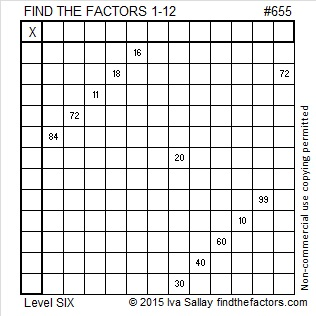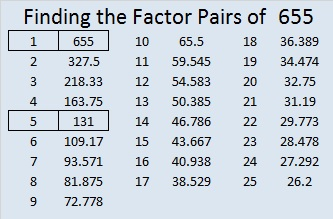# 655 and Level 6

655  is the hypotenuse of the Pythagorean triple 393-524-655. What is the greatest common factor of those three numbers?

655 is a leg in exactly three triples. One of them is primitive; the rest are not. Which is which?

• 655-1572-1703
• 655-42900-42905
• 655-214512-214513

Which of 655’s factors are the greatest common factors of each of the two that are not primitive?Print the puzzles or type the solution on this excel file: 12 Factors 2015-10-19

—————————————————————————————————

• 655 is a composite number.
• Prime factorization: 655 = 5 x 131
• The exponents in the prime factorization are 1 and 1. Adding one to each and multiplying we get (1 + 1)(1 + 1) = 2 x 2 = 4. Therefore 655 has exactly 4 factors.
• Factors of 655: 1, 5, 131, 655
• Factor pairs: 655 = 1 x 655 or 5 x 131
• 655 has no square factors that allow its square root to be simplified. √655 ≈ 25.592968.—————————————————————————————————